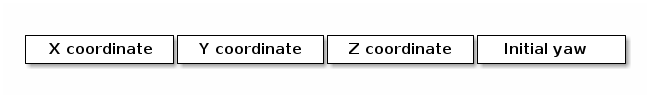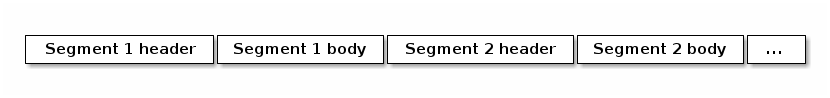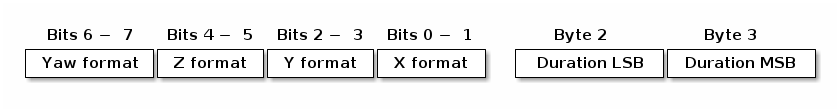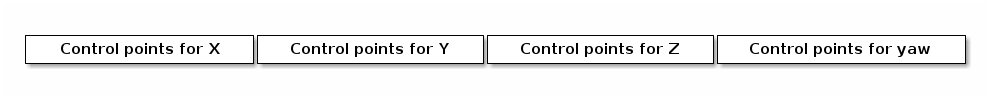# Trajectory formats

This document describes how the Crazyflie high-level commander stores trajectories in the internal trajectory memory.

Trajectories for the high-level commander may be stored in one of two formats, either as a sequence of raw 7th degree polynomials, or as a more space-efficient representation using the same polynomials in Bernstein form. In both cases, a single segment stores a duration and one polynomial for each of the X, Y, Z and yaw coordinates of the trajectory.

## Raw representation

The raw representation stores the coefficients of the polynomial describing the X coordinate of the trajectory first, starting with the constant term and ending with the 7th degree term, followed by the terms of the polynomials describing the Y, Z and yaw coordinates, and the duration of the segment itself in seconds. Each term and the duration is encoded as standard IEEE single-precision floats, meaning that a single segment requires 132 bytes: 8x4 floats for the X, Y, Z and yaw components per trajectory segment, plus one additional float per segment to store its duration. Given that the default size of the trajectory memory is 4 Kbytes, you can only store 31 segments.

## Compressed representation

The compressed representation was designed to be more space-efficient than the raw representation while still trying to maintain almost the same degree of accuracy as the raw representation.

The general guidelines of this representation are as follows:

• Durations are represented as milliseconds and are stored as signed 2-byte integers.

• Spatial coordinates (X, Y and Z) are represented as millimeters and are stored as signed 2-byte integers, meaning that the maximum spatial volume that this representation can cover is roughly 64m x 64m x 64m, assuming that the origin is at the center.

• Angles (for the yaw coordinate) are represented as 1/10th of degrees and are stored as signed 2-byte integers.

• Trajectory segments are described with varying degrees of Bézier curves, ranging from degree 0 (constant) to degree 7 (full 7D polynomial, as in the raw representation).

The compressed representation starts with the description of the starting point of the trajectory:This is then followed by data blocks describing the individual segments - one data block per segment:The header of a segment is three bytes long. The first byte describes how the X, Y, Z and yaw coordinates will be encoded in the body of the data block. The remaining two bytes contain the duration of the segment:For each of the X, Y, Z and yaw coordiates, there are two bits in the first byte of the header. 00 means that the coordinate is constant throughout the section (i.e. it is a zero-order Bézier curve). 01 means that the coordinate changes linearly (it is a Bézier curve of order 1). 10 means that the coordinate changes according to a cubic Bézier curve. 11 means that the coordinate changes according to a full 7th degree polynomial, expressed again as a 7th degree Bézier curve.

For instance, a header containing 0x0a 0xd0 0x07 means that the segment is 2000 milliseconds long (because 0x07d0 = 2000), and it will have a constant yaw and Z coordinate. Cubic Bézier curves will be used to describe the X and Y coordinates of the segment. (This is because 0x0a = 00 00 10 10 in binary).

Note that the choice of Bézier curves means that we need to store not the raw coefficients of the corresponding polynomials (which may be anywhere in the full 4-byte float range), but the coordinates of the control points of the Bézier curves, whose range is much more predictable and easier to represent with a fixed millimeter-scale quantization.

The body of the segment is then simply a concatenation of the control points for the X, Y, Z and yaw coordinates, omitting the first control point because it is always the same as the last control point of the previous segment. (That’s why we needed to store the starting point of the trajectory separately).Obviously, when the header specifies that a coordinate is constant, it means that we do not need to store the control points for that coordinate at all (there would be only a single control point, but we already know that it is the same as the last control point of the previous segment). For linear segments, we only need to store the end of the segment. For cubic Bézier segments, we need to store the first and second control point and the end of the segment. This can result in significant space savings if the trajectory consists mostly of linear segments and cubic Bézier curves, especially if the yaw does not change, or if the movement mostly occurs in the X-Y, X-Z or Y-Z plane. Note that cubic Bézier curves are enough to ensure C0, C1 and C2 continuity (in position, velocity and acceleration) for the trajectories.

Internally, whenever the high-level commander starts processing a trajectory segment that is encoded using the compressed representation, it converts the Bézier curve back into its raw polynomial representation. It means that most of the codebase only needs to work with raw 7th degree polynomials.

A downside of the compressed representation is that it is hard to play the trajectory backwards. The current implementation does not support reverse traversal at all.